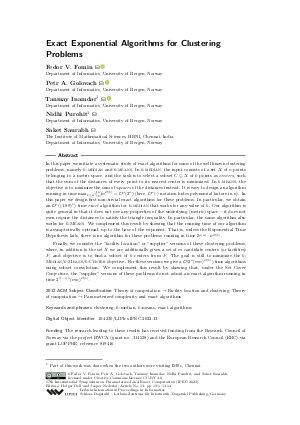Document# Exact Exponential Algorithms for Clustering Problems

### Authors Fedor V. Fomin, Petr A. Golovach, Tanmay Inamdar, Nidhi Purohit, Saket Saurabh## File

LIPIcs.IPEC.2022.13.pdf
• Filesize: 0.88 MB
• 14 pages

## Cite As

Fedor V. Fomin, Petr A. Golovach, Tanmay Inamdar, Nidhi Purohit, and Saket Saurabh. Exact Exponential Algorithms for Clustering Problems. In 17th International Symposium on Parameterized and Exact Computation (IPEC 2022). Leibniz International Proceedings in Informatics (LIPIcs), Volume 249, pp. 13:1-13:14, Schloss Dagstuhl - Leibniz-Zentrum für Informatik (2022)
https://doi.org/10.4230/LIPIcs.IPEC.2022.13

## Abstract

In this paper we initiate a systematic study of exact algorithms for some of the well known clustering problems, namely k-MEDIAN and k-MEANS. In k-MEDIAN, the input consists of a set X of n points belonging to a metric space, and the task is to select a subset C ⊆ X of k points as centers, such that the sum of the distances of every point to its nearest center is minimized. In k-MEANS, the objective is to minimize the sum of squares of the distances instead. It is easy to design an algorithm running in time max_{k ≤ n} {n choose k} n^𝒪(1) = 𝒪^*(2ⁿ) (here, 𝒪^*(⋅) notation hides polynomial factors in n). In this paper we design first non-trivial exact algorithms for these problems. In particular, we obtain an 𝒪^*((1.89)ⁿ) time exact algorithm for k-MEDIAN that works for any value of k. Our algorithm is quite general in that it does not use any properties of the underlying (metric) space - it does not even require the distances to satisfy the triangle inequality. In particular, the same algorithm also works for k-Means. We complement this result by showing that the running time of our algorithm is asymptotically optimal, up to the base of the exponent. That is, unless the Exponential Time Hypothesis fails, there is no algorithm for these problems running in time 2^o(n)⋅n^𝒪(1). Finally, we consider the "facility location" or "supplier" versions of these clustering problems, where, in addition to the set X we are additionally given a set of m candidate centers (or facilities) F, and objective is to find a subset of k centers from F. The goal is still to minimize the k-Median/k-Means/k-Center objective. For these versions we give a 𝒪(2ⁿ (mn)^𝒪(1)) time algorithms using subset convolution. We complement this result by showing that, under the Set Cover Conjecture, the "supplier" versions of these problems do not admit an exact algorithm running in time 2^{(1-ε) n} (mn)^𝒪(1).

## Subject Classification

##### ACM Subject Classification
• Theory of computation → Facility location and clustering
• Theory of computation → Parameterized complexity and exact algorithms
##### Keywords
• clustering
• k-median
• k-means
• exact algorithms

## Metrics

• Access Statistics
• Total Accesses (updated on a weekly basis)
0

## References

1. Pankaj K Agarwal and Cecilia Magdalena Procopiuc. Exact and approximation algorithms for clustering. Algorithmica, 33(2):201-226, 2002.2. Andreas Björklund, Thore Husfeldt, and Mikko Koivisto. Set partitioning via inclusion-exclusion. SIAM Journal on Computing, 39(2):546-563, 2009.3. Jérémie Chalopin and Daniël Paulusma. Packing bipartite graphs with covers of complete bipartite graphs. Discret. Appl. Math., 168:40-50, 2014. URL: https://doi.org/10.1016/j.dam.2012.08.026.
4. Vincent Cohen-Addad, Anupam Gupta, Amit Kumar, Euiwoong Lee, and Jason Li. Tight fpt approximations for k-median and k-means. arXiv preprint, 2019. URL: http://arxiv.org/abs/1904.12334.
5. Marek Cygan, Holger Dell, Daniel Lokshtanov, Dániel Marx, Jesper Nederlof, Yoshio Okamoto, Ramamohan Paturi, Saket Saurabh, and Magnus Wahlström. On problems as hard as cnf-sat. ACM Trans. Algorithms, 12, 2016.6. Marek Cygan, Fedor V Fomin, Łukasz Kowalik, Daniel Lokshtanov, Dániel Marx, Marcin Pilipczuk, Michał Pilipczuk, and Saket Saurabh. Parameterized algorithms, volume 5(4). Springer, 2015.7. Fedor V Fomin, Serge Gaspers, Daniel Lokshtanov, and Saket Saurabh. Exact algorithms via monotone local search. Journal of the ACM (JACM), 66(2):1-23, 2019.8. Fedor V Fomin, Fabrizio Grandoni, and Dieter Kratsch. A measure & conquer approach for the analysis of exact algorithms. Journal of the ACM (JACM), 56(5):1-32, 2009.9. M. R. Garey and David S. Johnson. Computers and Intractability: A Guide to the Theory of NP-Completeness. W. H. Freeman, 1979.10. Wen-Lian Hsu and George L Nemhauser. Easy and hard bottleneck location problems. Discrete Applied Mathematics, 1(3):209-215, 1979.11. Russell Impagliazzo and Ramamohan Paturi. On the complexity of k-sat. J. Comput. Syst. Sci., 9:367-375, 2001.12. Yoichi Iwata. A faster algorithm for dominating set analyzed by the potential method. In International Symposium on Parameterized and Exact Computation, pages 41-54. Springer, 2011.13. Edmonds Jack. Paths, trees, and flowers. Canadian Journal of Mathematics, 17:449-467, 1965.14. Kamal Jain and Vijay V Vazirani. Approximation algorithms for metric facility location and k-median problems using the primal-dual schema and lagrangian relaxation. Journal of the ACM (JACM), 48(2):274-296, 2001.15. David G. Kirkpatrick and Pavol Hell. On the complexity of general graph factor problems. SIAM J. Comput., 12(3):601-609, 1983. URL: https://doi.org/10.1137/0212040.
16. Dieter Kratsch and FV Fomin. Exact exponential algorithms. Springer-Verlag Berlin Heidelberg, 2010.17. Stuart Lloyd. Least squares quantization in pcm. IEEE transactions on information theory, 28(2):129-137, 1982.18. Daniel Lokshtanov, Dániel Marx, and Saket Saurabh. Lower bounds based on the exponential time hypothesis. Bull. EATCS, 105:41-72, 2011.19. James MacQueen et al. Some methods for classification and analysis of multivariate observations. In Proceedings of the fifth Berkeley symposium on mathematical statistics and probability, volume 1(14), pages 281-297. Oakland, CA, USA, 1967.20. Hugo Steinhaus et al. Sur la division des corps matériels en parties. Bull. Acad. Polon. Sci, 1(804):801, 1956.21. Ola Svensson, Jakub Tarnawski, and László A Végh. A constant-factor approximation algorithm for the asymmetric traveling salesman problem. Journal of the ACM (JACM), 67(6):1-53, 2020.22. Vera Traub and Jens Vygen. An improved approximation algorithm for atsp. In Proceedings of the 52nd annual ACM SIGACT symposium on theory of computing, pages 1-13, 2020.23. Wikipedia. Geometric median - Wikipedia, the free encyclopedia. http://en.wikipedia.org/w/index.php?title=Geometric%20median&oldid=1061179886, 2022. [Online; accessed 21-April-2022].
24. Wikipedia. Weber problem - Wikipedia, the free encyclopedia. http://en.wikipedia.org/w/index.php?title=Weber%20problem&oldid=916663348, 2022. [Online; accessed 21-April-2022].
25. Gerhard J Woeginger. Exact algorithms for np-hard problems: A survey. In Combinatorial optimization - eureka, you shrink!, pages 185-207. Springer, 2003.X

Feedback for Dagstuhl Publishing